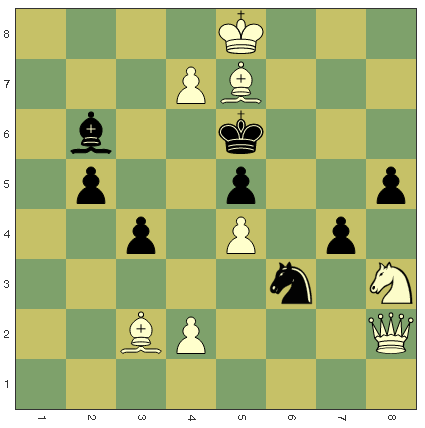# Mate!

Logic Level 4Consider the positional set up of a game. It is white's turn to move. White can checkmate black in just two moves, can you find the first move to force a checkmate?

If the first move can be written as $(x_1,y_1) , (x_2,y_2)$, where $x_1,y_1$ are the coordinates (according to how it is marked above) of the moved piece's initial position and $x_2,y_2$ are the coordinates of the position of the piece after the move, evaluate $(x_1+y_1) \times (x_2+y_2)$

×

Problem Loading...

Note Loading...

Set Loading...